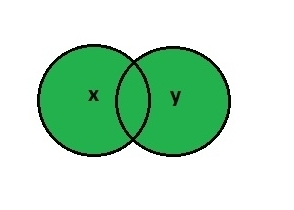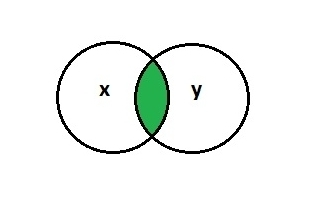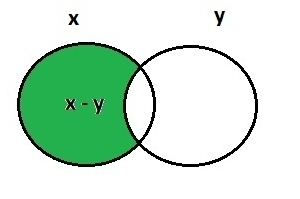# Ruby – Set Operations

• Last Updated : 03 Jul, 2020

The set is an unordered collection with no duplicate items. Like other languages, Ruby also provides a set class that represents the mathematical notion of a set. Here, we will discuss the basic set operations, i.e., Union, Intersection, and Difference.

### Union

In Ruby, the union takes two objects like arrays, vectors, hashes, etc. as arguments and results in a third object which contains all the elements from both the objects and removes duplicate elements. You can perform a union between two arrays using the pipe operator(|).

Syntax:
x | y

Parameters: x and y are the sequence items of objects.

For Example:

`[1, 2, 1, 2, 3, 5, 7] | [1, 2, 3, 4] #=> [1, 2, 3, 4, 5, 7]`Example 1:

 `# Ruby program to illustrate the union`` ` `# Array``arr_1 = [ ``2``, ``4``, ``5``, ``6``, ``7``, ``9``, ``1``, ``2``, ``4` `] ``arr_2 = [ ``2``, ``4``, ``5``, ``6``, ``9``, ``8``, ``0``, ``3``, ``3` `] `` ` `# Union `` ``result = arr_1 | arr_2``  ` ` ``# Display result`` ``puts ``"#{result}"`

Output:

`[2, 4, 5, 6, 7, 9, 1, 8, 0, 3]`

Example 2:

 `# Ruby program to illustrate Union of arrays``  ` `class` `MultiSet``  ``attr_accessor ``:set``  ``def` `initialize(set)``    ``@set` `= set``  ``end`` ` `# Union``  ``def` `|(other)``    ``@set` `| other.set``  ``end``end``  ` `x = MultiSet.``new``([ ``1``, ``1``, ``2``, ``2``, ``3``, ``4``, ``5``, ``6``, ``7``, ``8` `])``y = MultiSet.``new``([ ``1``, ``3``, ``5``, ``6``, ``8` `])``  ` `p x | y `

Output:

`[1, 2, 3, 4, 5, 6, 7, 8]`

### Intersection

In Ruby, intersection takes two objects like arrays, vectors, hashes, etc. as arguments and results in a third object which contains elements that are common in both the objects and remove duplicates. The order in the intersection is preserved from the original array. You can perform intersection either by using & operator or by using the intersection() function.

Syntax:
x & y

Parameters: x and y are the sequence items of objects.

For Example:

`[ 'a', 'b', 'b', 'z' ] & [ 'a', 'b', 'c' ,'z']   #=> [ 'a', 'b' ,'z']`Example 1:

 `# Ruby program to illustrate the intersection method `` ` `# requires the set ``require ``"set"`` ` `x = Set.``new``([ ``1``, ``2``, ``3``, ``4` `])``y = Set.``new``([ ``1``, ``2``, ``4` `])`` ` `# Intersection method used ``res = x.intersection(y) `` ` `# Prints the res ``puts res`

Output:

`Set: {1, 2, 4}`

Example 2:

 `# Ruby program to illustrate intersection of arrays``class` `MultiSet``  ``attr_accessor ``:set``  ``def` `initialize(set)``    ``@set` `= set``  ``end`` ` `# Intersection``  ``def` `&(other)``    ``@set` `& other.set``  ``end``     ` `x = MultiSet.``new``([ ``1``, ``1``, ``2``, ``2``, ``3``, ``4``, ``5``, ``6``, ``7``, ``8` `])``y = MultiSet.``new``([ ``1``, ``3``, ``5``, ``6``, ``8` `])``  ` `p x & y ``end`

Output:

`[1, 3, 5, 6, 8]`

### Difference

In Ruby, difference takes two objects like arrays, vectors, hashes, etc. as arguments and returns the difference of both the objects. You can perform difference either by using – operator or by using the difference() function.

Syntax:
x – y

Parameters: x and y are the sequence items of objects.

For Example:

`[ 22, 45, 89, 76 ] - [ 22, 22, 89, 79 ]   #=> `Example 1:

 `# Ruby program to illustrate the difference method `` ` `# requires the set ``require ``"set"`` ` `x = Set.``new``([ ``1``, ``2``, ``3``, ``4` `])``y = Set.``new``([ ``1``, ``2``, ``4``, ``5` `])`` ` `# Difference method used ``res = x.difference(y) `` ` `# Prints the res ``puts res`

Output:

`Set: {3}`

Example 2:

 `# Ruby program to illustrate difference of arrays``class` `MultiSet``  ``attr_accessor ``:set``  ``def` `initialize(set)``    ``@set` `= set``  ``end`` ` `# Difference``  ``def` `-(other)``    ``@set` `- other.set``  ``end`` ` `x = MultiSet.``new``([ ``1``, ``1``, ``2``, ``2``, ``3``, ``4``, ``5``, ``6``, ``7``, ``8` `])``y = MultiSet.``new``([ ``1``, ``3``, ``5``, ``6``, ``8` `])``  ` `p x - y ``end`

Output:

`[2, 2, 4, 7]`

My Personal Notes arrow_drop_up